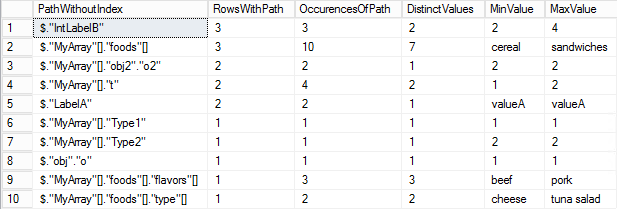# Querying Json Recursively in Azure SQL Database

This post is days old.

Dealing with JSON data in SQL Database introduces several challenges. This post addresses querying json data stored in SQL Database that is semi structured or of an unknown structure.

Let’s create some sample data:

``````drop table if exists t;
go
create table t(Id int identity primary key, json nvarchar(max))
insert t(json)
values('{
"LabelA": "valueA",
"IntLabelB": 2,
"MyArray":[
{"Type1":1,"foods":["hot dogs","peas"]},
{"Type2":2,"foods":["french toast","cereal"]}
]
}'),('{
"LabelA": "valueA",
"IntLabelB": 2,
"obj":{"o":1},
"MyArray":[
{"t":1,"foods":["hotdogs",{"flavors":["pork","beef","Chicken"]}]},
{"t":2,"foods":["cereal","eggs"],"obj2":{"o2":2}}
]
}'),('{
"IntLabelB": 4,
"MyArray":[
{"t":2,"foods":["cereal","eggs"],"obj2":{"o2":2}}
]
}');
go
``````

We can use `json_query` (which only selects valid json) and `json_value` (which only select a scalar), to build a query. This requires an undestanding of the path to the data we want:

``````select [LabelA]=json_value(t.json,'\$.LabelA'),
[IntLabelB]=json_value(t.json,'\$.IntLabelB'),
[MyArray_index0_t]=json_value(t.json,'\$.MyArray.t'),
[MyArray_index1_foods_json]=json_query(t.json,'\$.MyArray.foods')
from t;
``````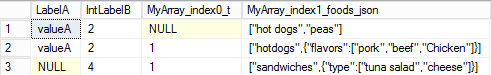Using this approach the json structure is highly relevant; The query reads specific items from precise paths. This could work to query a few important parts of json objects, or read many columns from highly structured data. It may not work well to explore a large collection of data or to query semi structured data.

We can also use `openjson` to select as key-value data:

``````select t.Id,j.*
from t
outer apply openjson(t.json) j;
``````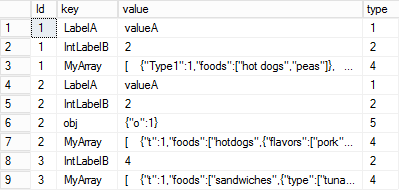However, `openjson` does not handle complex json, other than returning `[value]="some json"` for nested arrays and objects.

Instead, we can expanded nested json data using multiple `openjson` for each nesting of data!:

``````select t.Id,level1.*,level2.*,level3.*,level4.*
from t
outer apply openjson(t.json) level1
outer apply (select * from openjson(level1.[value]) where level1.[type]>3) level2
outer apply (select * from openjson(level2.[value]) where level2.[type]>3) level3
outer apply (select * from openjson(level3.[value]) where level3.[type]>3) level4
where id=1;
``````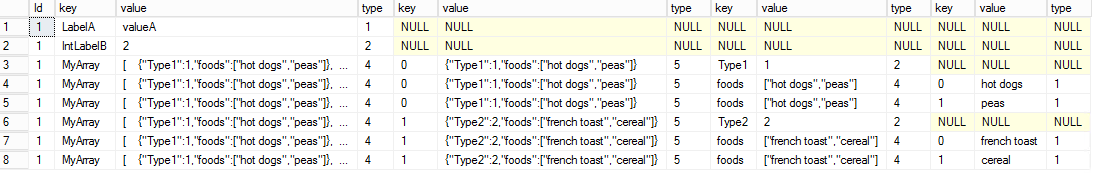This results in the data we are looking for, although it is a bit difficult to read (or query).

Next, we can formalize the above query idea, using a recursive query, clean up data/columns, and return json path information.

``````drop view if exists viewJsonData;
go
create view viewJsonData
as
with r([Id],[Path],[PathWithoutIndex],[key],[value],[type])as(
select [Id],[Path]=cast(concat('\$."',[key],'"') as nvarchar(max)),[PathWithoutIndex]=cast(concat('\$."',[key],'"') as nvarchar(max)),[key],[value],[type]
from t
outer apply openjson(t.[json])
union all
select [Id],[Path]=cast((case when r.[type]=4 then concat(r.[Path],'[',k.[key],']') else concat(r.[Path],'."',k.[key],'"') end) as nvarchar(max)),
[PathWithoutIndex]=cast((case when r.[type]=4 then concat(r.[PathWithoutIndex],'[]') else concat(r.[PathWithoutIndex],'."',k.[key],'"') end) as nvarchar(max)),
k.[key],k.[value],k.[type]
from r
outer apply openjson(r.[value]) k
where r.[type]>3
)
select *
from r
where [type]<4;		/* Type 4 and 5 are the json objects that we recursively open, so those data elements would also exist in the query results as type<4 */
go
``````

This view should provide reasonably efficient querying when looking at individual rows (specified by the Primary key). The number of recursions is relative to the depth of the json data. This approach would also be helpful for exploring collections of json data to identify where data is.

Sample usage 1: reading all of the json data from a single row

``````select * from viewJsonData where Id=3;
``````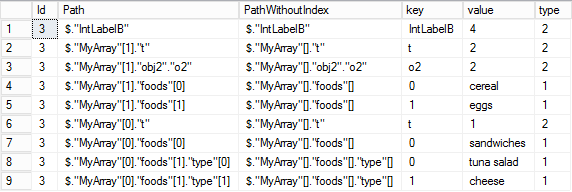Sample usage 2: exploring unfamiliar data

``````select
[PathWithoutIndex],
[RowsWithPath]=count(distinct Id),
[OccurencesOfPath]=count(1),
[DistinctValues]=count(distinct value),
[MinValue]=min(value),
[MaxValue]=max(value)
from viewJsonData
group by [PathWithoutIndex]
order by 2 desc;
``````